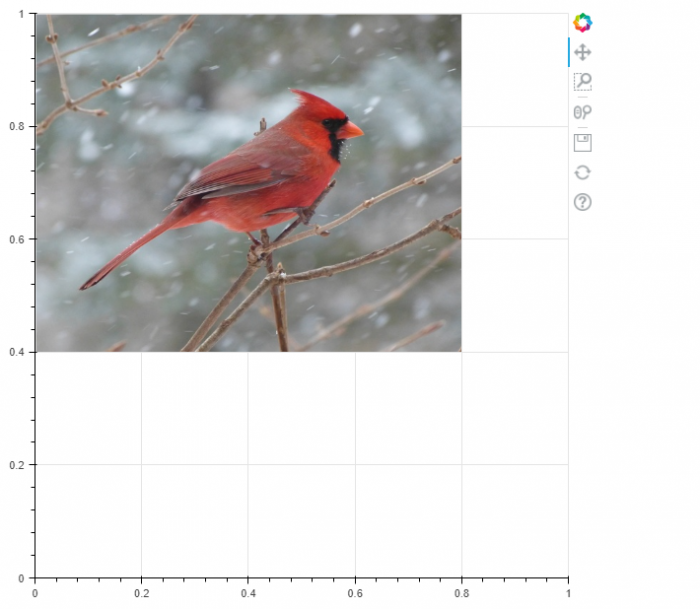# How to embed an interactive Matplotlib plot on a webpage?

To show a plot on a webpage such that the plot could be interactive, we can take the following steps −

• Install Bokeh and import figure, show, and output_file.
• Configure the default output state to generate the output saved to a file when:func:'show' is called.
• Create a new Figure for plotting.
• Render the images loaded from the given URLs.
• Immediately display a Bokeh object or application.

## Example

from bokeh.plotting import figure, show, output_file

output_file('image.html')
p = figure(x_range=(0, 1), y_range=(0, 1))

p.image_url(url=['bird.jpg'], x=0, y=1, w=0.8, h=0.6)
show(p)

## Output

When we execute the code, it will show the following image on your default browser.You can move the image around on the browser, as the plot is interactive